# Common Core: 7th Grade Math : Understand Distances Between Numbers on a Number Line: CCSS.Math.Content.7.NS.A.1b

## Example Questions

← Previous 1 3 4

### Example Question #2 : Arithmetic

Add: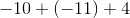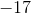Explanation:

Simply the signs before solving. A positive sign multiplied with a negative sign will convert the sign to a negative, and a negative multiplied with a negative will convert the sign to a positive.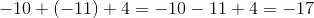### Example Question #31 : The Number System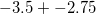is equal to which of the following?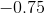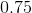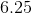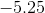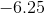Explanation:

This is a straightforward problem. Remember that when adding a negative number, you are actually subtracting: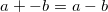Be sure to remember that the first number is also negative, meaning we are subtracting a number from a negative number: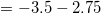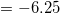### Example Question #1 : How To Add Fractions

Evaluate: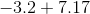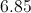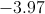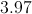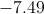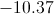Explanation:

The sum of two numbers of unlike sign is the difference of their absolute values, with the sign of the "dominant" number (the positive number here) affixed: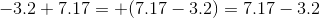Subtract vertically by aligning the decimal points, making sure you append the 3.2 with a placeholder zero: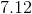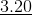This is the correct choice.

### Example Question #21 : Negative Numbers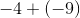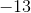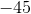Explanation:

When we add a negative number, the sign turns negative. Since we are adding two negative numbers, we treat this as an addition problem and add a minus sign in the end.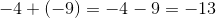### Example Question #1 : Understand Distances Between Numbers On A Number Line: Ccss.Math.Content.7.Ns.A.1b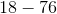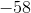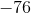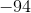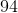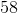Explanation:

When dealing with negative numbers, let's see which number is greater.is greater thanand is negative so the answer is negative. We treat this as a subtraction problem.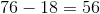. Because our answer should be negative, the correct answer is### Example Question #1 : Understand Distances Between Numbers On A Number Line: Ccss.Math.Content.7.Ns.A.1b

Solve: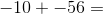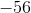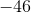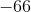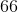Explanation:

Remember that adding a negative number is the same as subtracting that same number if it were positive.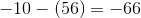### Example Question #41 : The Number System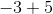Explanation:

Sinceis greater thanand is positive, our answer is positive. We treat as a subtraction problem. Answer is.

### Example Question #8 : Understand Distances Between Numbers On A Number Line: Ccss.Math.Content.7.Ns.A.1b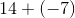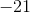Explanation:

When a plus and minus sign meet, the sign is negative. The difference is.

### Example Question #41 : The Number System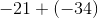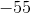Explanation:

When a plus and a minus meet, the sign is negative. When adding two negatie numbers, we treat as addition and add the minus sign in the end. Answer is.

### Example Question #1 : Understand Distances Between Numbers On A Number Line: Ccss.Math.Content.7.Ns.A.1b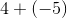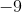Explanation:

When a plus and minus sign meet, we have a minus sign.

Sinceis greater thanand is negative, our answer is negative.

We treat as a normal subtraction.

Answer is.

← Previous 1 3 4

### All Common Core: 7th Grade Math Resources